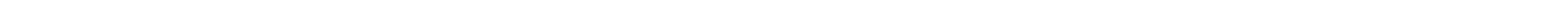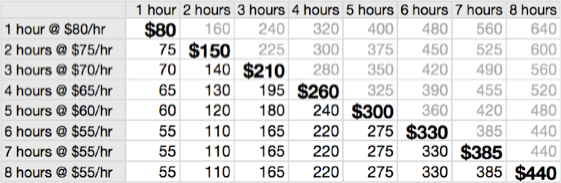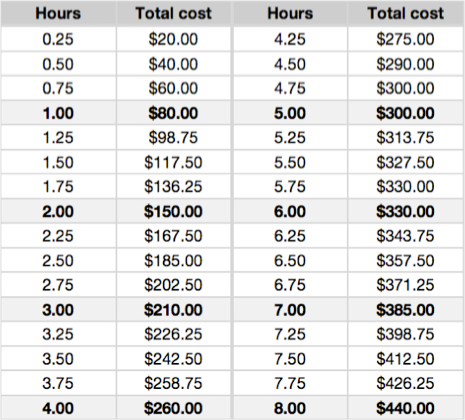The large bold numbers represent the total cost of labor for that many hours, while the gray numbers in the upper right 'triangle' represent the total cost of labor if the hourly rate had not declined. For example, if the job only takes an hour, then the cost of labor is \$80. However, if the job takes 4 hours, the cost of labor is \$260 (that’s 4 x \$65), instead of \$320 (which is 4 x \$80). That's a "savings" of \$60 in labor over the 1-hour rate. If the job takes 8 hours, the cost of labor is \$440 (that's 8 x \$55), a “savings” of \$120 over the 4-hour rate and a "savings" of \$200 over the 1-hour rate. Basically, the longer you hire me, the less you pay per hour.

If you are wondering why I charge like this, that’s answered on the